Home Plan For College Saving for College The Rule of 72: Calculate How Long It Will Take an Investment to Double in Value

# The Rule of 72: Calculate How Long It Will Take an Investment to Double in Value

The Rule of 72 is a simple rule of thumb for estimating how many years it will take an investment to double in value at a particular interest rate, compounded annually. To calculate an estimate of the doubling time, divide 72 by the interest rate. For example, for an investment earning 4%, it will take about 18 years (72/4) for the investment to double in value.

The Rule of 72 can also be used to estimate the interest rate necessary to double the value of an investment in a particular number of years. For example, to double an investment in 6 years requires an interest rate of about 72/6 = 12 percent.

Find & Compare Private Student Loans for Your School:

CONTENTS:

## Accuracy of the Rule of 72

The Rule of 72 is an approximation, not entirely accurate. For example, the actual doubling time for an investment with a 4% annual return is log (2) / log (1+4%) = 17.7 years, not 18 years. The actual interest rate required to double an investment in 6 years is 21/6 - 1 = 12.2%, not 12%.

But the Rule of 72 is reasonably accurate, especially for interest rates between 6% and 10%.

A rule of 73 would yield a more accurate result, but the Rule of 72 works well because 72 is evenly divisible by many potential small divisors: 2, 3, 4, 6, 8, 9, 12, 18, 24, 36.

## Similar Rules of Thumb

One can derive several similar rules for other multiple increases in the investment value, as illustrated in the following table of rules like the Rule of 72.

Increase in Investment Value Rule Based on Product of Time and Rate
Double (2x) 72
Triple (3x) 120
Quintuple (5x) 160 or 168
Sextuple (6x) 180 or 192
Septuple (7x) 196 or 200
Octuple (8x) 210 or 216

One could substitute a rule of 119 for the Rule of 120 for calculating the time to triple an investment. Normally, one would not choose 119 for a rule, since it is the product of two prime numbers, 7 and 17. But 17 is the typical number of years from birth to matriculation in college and college costs tend to triple over any 17-year period, making the Rule of 119 useful for college savings. One might consider 128 (age 16) and 108 or 126 (age 18) for similar reasons. Or one could just remember that an interest rate of about 7% is required for a lump sum investment to triple from birth to enrollment in college.

To estimate the doubling time for daily compounding, as opposed to annual compounding, use the Rule of 69.

To estimate the number of years for an inflation rate to cause the value of an investment to drop in half, use the Rule of 70. Just divide 70 by the inflation rate. For example, a 2% inflation rate will cut the value of an investment in half in about 35 years (70/2).

A related rule of thumb calculates an underestimate of the total interest paid on a loan with a level amortization. Let R be the interest rate and B the loan balance. A straight-line approximation assumes that the annual interest will average to about the average of the interest on the first and last years of the repayment term, which should be about B and zero. This yields R⋅B / 2 as the average annual interest.

Multiply this by N, the number of years in the repayment term, yields (R⋅B / 2) ⋅ N as an estimate of the total interest paid. For example, the total interest paid over the life of a \$10,000 loan with a 10% interest rate and a 10-year term is 10% x \$10,000 / 2 x 10 = \$5,000. This is slightly less than the actual total, which is \$5,858. The estimate is always slightly lower than the actual figure because a linear approximation is less than a level amortization curve, which is convex in shape. To calculate an estimate of the total payments over the life of the loan, add in the loan balance, yielding (R⋅B / 2) ⋅ N + B. For example, using the same \$10,000 loan example with a 10% interest rate and 10-year repayment term, the total payments over the life of the loan is slightly more than 10% x \$10,000 / 2 x 10 + \$10,000 = \$15,000.

## Derivation of the Rules

The formula relating interest rates and actual doubling time is straightforward. Let R be the annual percentage return on investment and N the number of years required to increase the investment value by a factor of M. Then these variables are related by the formula: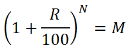A rule of thumb, however, should involve simpler calculations than exponentiation. There are several approaches to approximating the formula in ways that make it easier for people to remember and use.

### Logarithm Approach

One approach involves taking the logarithm of both sides of the equation (1 + R/100)N = M. This yields N ⋅ log (1 + R/100) = log (M). Solving for N ⋅ R yields N ⋅ R = R / log (1 + R/100) ⋅ log (M).

This formula yields 71.4 for R = 6 and M = 2, increasing to 72.7 for R = 10. For M = 3, this yields a product of 113.1 for R = 6 and 115.3 for R = 10. For M = 4, this yields a product of 142.7 for R = 6 and 145.5 for R = 10. The products do not vary much, but one could use an average of the ratios or a ratio of the averages for a range of R values. Since this approach uses the equivalent of the actual formula instead of approximating it, the products are more precise.

In each case, one picks the nearest whole number with many low divisors for ease of calculation.

### Exponentiation Expansion Approach

Another approach involves expanding the exponentiation: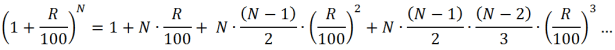This expansion can be approximated by dropping the higher order terms involving powers of 3 and beyond.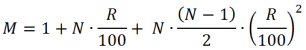Solving for N ⋅ (R/100) yields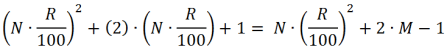One can discard the N ⋅ (R/100)2 term because it is small and does not contribute much to the formula. This simplification makes it easier to factor the quadratic equation, yielding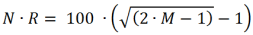For M = 2, we have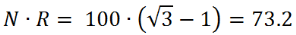from which one gets the Rule of 72 for doubling time by rounding to the nearest number with many factors. For M = 3, we have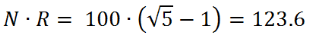from which one gets the Rule of 120 for the time to triple the value of an investment. For M = 4, we have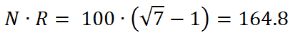from which one gets the Rule of 144 for the time to quadruple the value of an investment. The Rule of 144 is also double the Rule of 72.